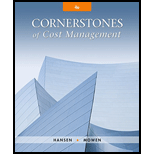# Refer to the data in Exercise 9.15. Required: 1. Compute overhead variances using a two-variance analysis. 2. Compute overhead variances using a three-variance analysis. 3. Illustrate how the two- and three-variance analyses are related to the four-variance analysis. Oerstman, Inc., uses a standard costing system and develops its overhead rates from the current annual budget. The budget is based on an expected annual output of 120,000 units requiring 480,000 direct labor hours. (Practical capacity is 500,000 hours.) Annual budgeted overhead costs total $787,200, of which$556,800 is fixed overhead. A total of 119,400 units using 478,000 direct labor hours were produced during the year. Actual variable overhead costs for the year were $230,600, and actual fixed overhead costs were$556,250. Required: 1. Compute the fixed overhead spending and volume variances. How would you interpret the spending variance? Discuss the possible interpretations of the volume variance. Which is most appropriate for this example? 2. Compute the variable overhead spending and efficiency variances. How is the variable overhead spending variance like the price variances of direct labor and direct materials? How is it different? How is the variable overhead efficiency variance related to the direct labor efficiency variance?### Cornerstones of Cost Management (C...

4th Edition
Don R. Hansen + 1 other
Publisher: Cengage Learning
ISBN: 9781305970663### Cornerstones of Cost Management (C...

4th Edition
Don R. Hansen + 1 other
Publisher: Cengage Learning
ISBN: 9781305970663

#### Solutions

Chapter 9, Problem 16E
Textbook Problem

## Expert Solution

### Want to see the full answer?

Check out a sample textbook solution.

### Want to see this answer and more?

Experts are waiting 24/7 to provide step-by-step solutions in as fast as 30 minutes!*

*Response times vary by subject and question complexity. Median response time is 34 minutes and may be longer for new subjects.